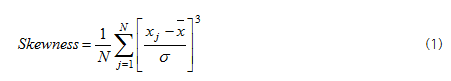Skewness, defined as the third moment value of signal, shows the asymmetrical degree of probability distribution graph.If this value is positive one, the peak is generally inclined to the positive direction in the graph. In the case of negative value, the peak of the probability distribution graph is inclined to the negative direction. Therefore, this value shows how probability distribution of data is different from normal distribution.By use of the skewness analysis method you can analyze the skewness of each channel from the data and have it displayed on a display window. This case is to analyze the skewness for the whole data. On the other hand, if you wish to analyze the skewness changing with time, you can use the moving window analysis together. Select the target data from the analysis window first and then click Moving Window analysis icon in the analysis tool bar. And a window setting dialog box as shown below will pop up. In this dialog box, you can configure the window value and the overlap ratio of your choice; the former means the range of the data, while the latter represents the degree of overlap among the data. The window value and the overlap ratio are explained in the analysis method of Moving Window in detail. Please refer to it!